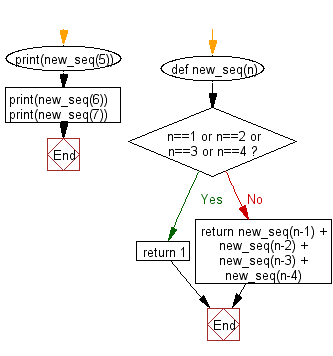﻿ Python: Find the Nth member of the sequence - w3resource

# Python: Find the Nth member of the sequence

## Python Basic - 1: Exercise-22 with Solution

Write a Python program to create a sequence where the first four members of the sequence are equal to one. Each successive term of the sequence is equal to the sum of the four previous ones. Find the Nth member of the sequence.

Sample Solution:

Python Code:

``````def new_seq(n):
if n==1 or n==2 or n==3 or n==4:
return 1
return new_seq(n-1) + new_seq(n-2) + new_seq(n-3) + new_seq(n-4)
print(new_seq(5))
print(new_seq(6))
print(new_seq(7))
``````

Sample Output:

```4
7
13
```

Flowchart:Python Code Editor:

Have another way to solve this solution? Contribute your code (and comments) through Disqus.

What is the difficulty level of this exercise?

Test your Programming skills with w3resource's quiz.

﻿